# Printable Time Tables Multiplication Chart 20ChartApr 01, 2021By PrintableeNo Comment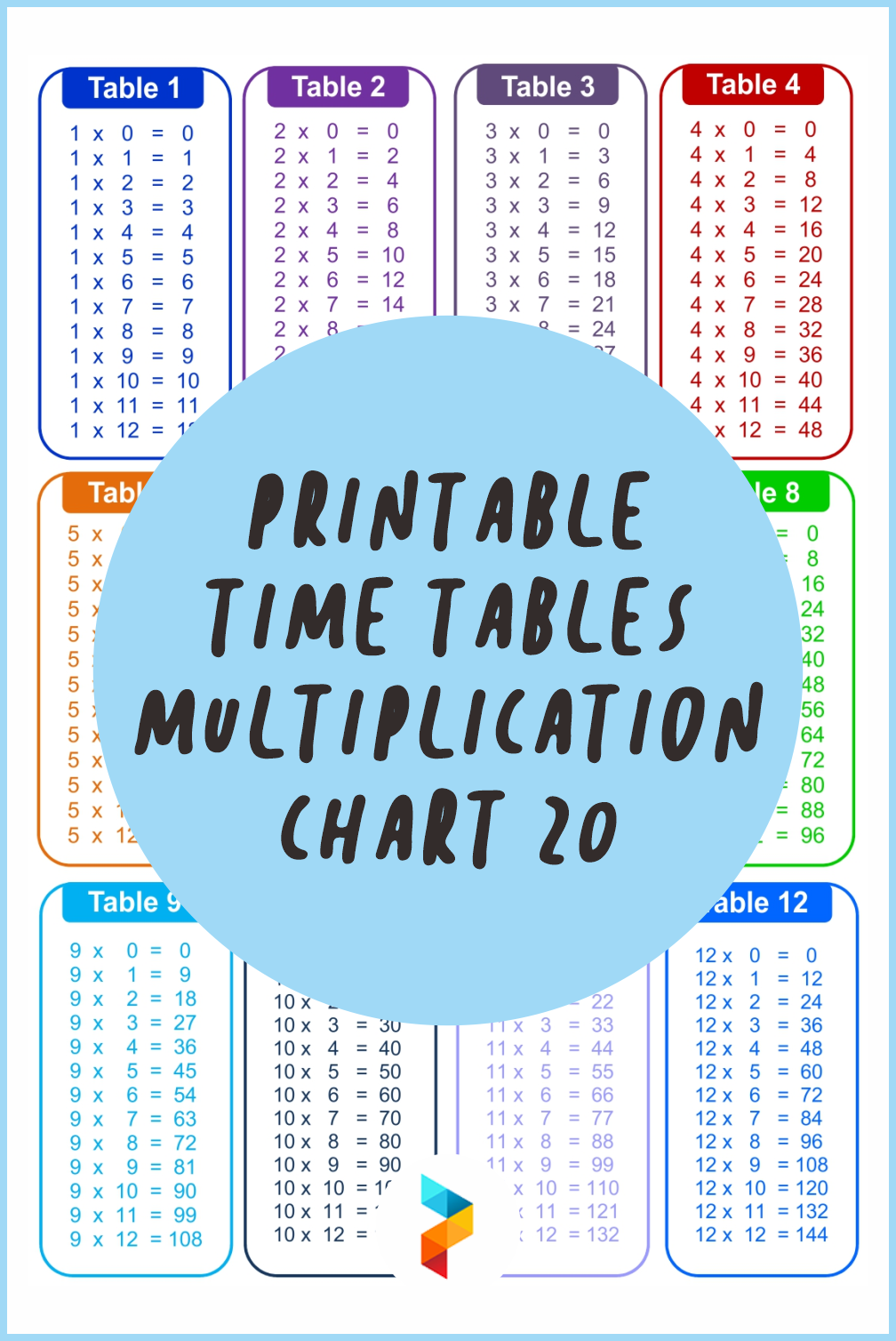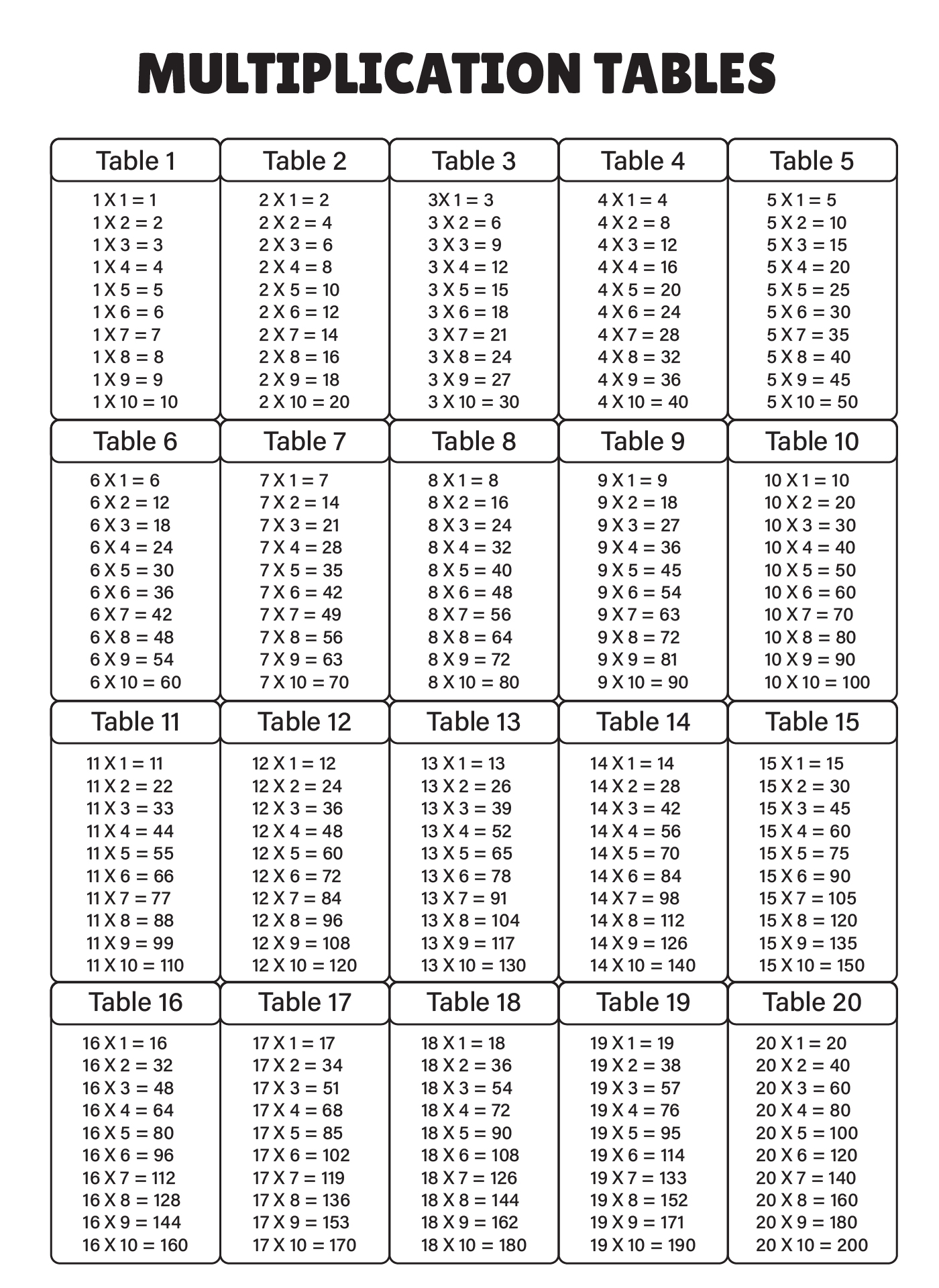### What are the 20 times tables?

When it comes to learning multiplication, there is a device that helps us to understand the concept of this kind of operation. Yes, we may have heard about time tables multiplication chart 20 before. This aims to learn about multiplication, specifically learning the numbers that are multiplied by twenty. The numbers usually come in 1 to 9 numbers. However, there also comes several numbers more than nine that are multiplied by 20. Usually, the amount of numbers to be multiplied is also 20. This means that the last number to be multiplied inside the chart is 20x20.

### At what age should a child know times tables?

Kids can start to learn 20 time tables with tables multiplication chart 20 when they are in the second year in elementary school. We may have seen that kids recite the multiplications when they are learning skipping counts. For instance, when they name loudly "two, four, six, eight, ten" and many more, they are actually introduced with multiplication.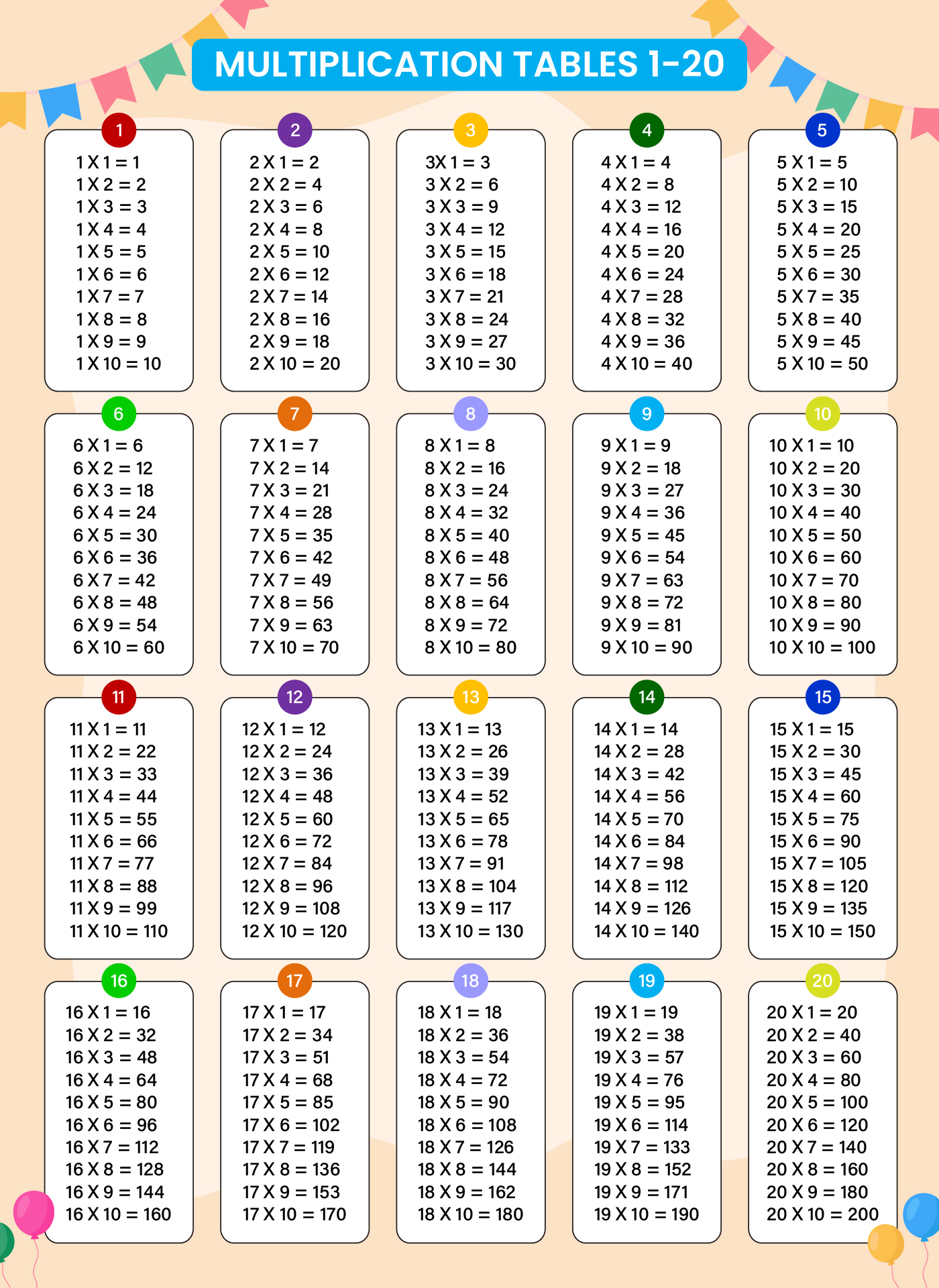We also have more printable chart you may like:
Printable Multiplication Chart 100 X
Printable Number Chart 1 -100
Printable Number Chart 1 -120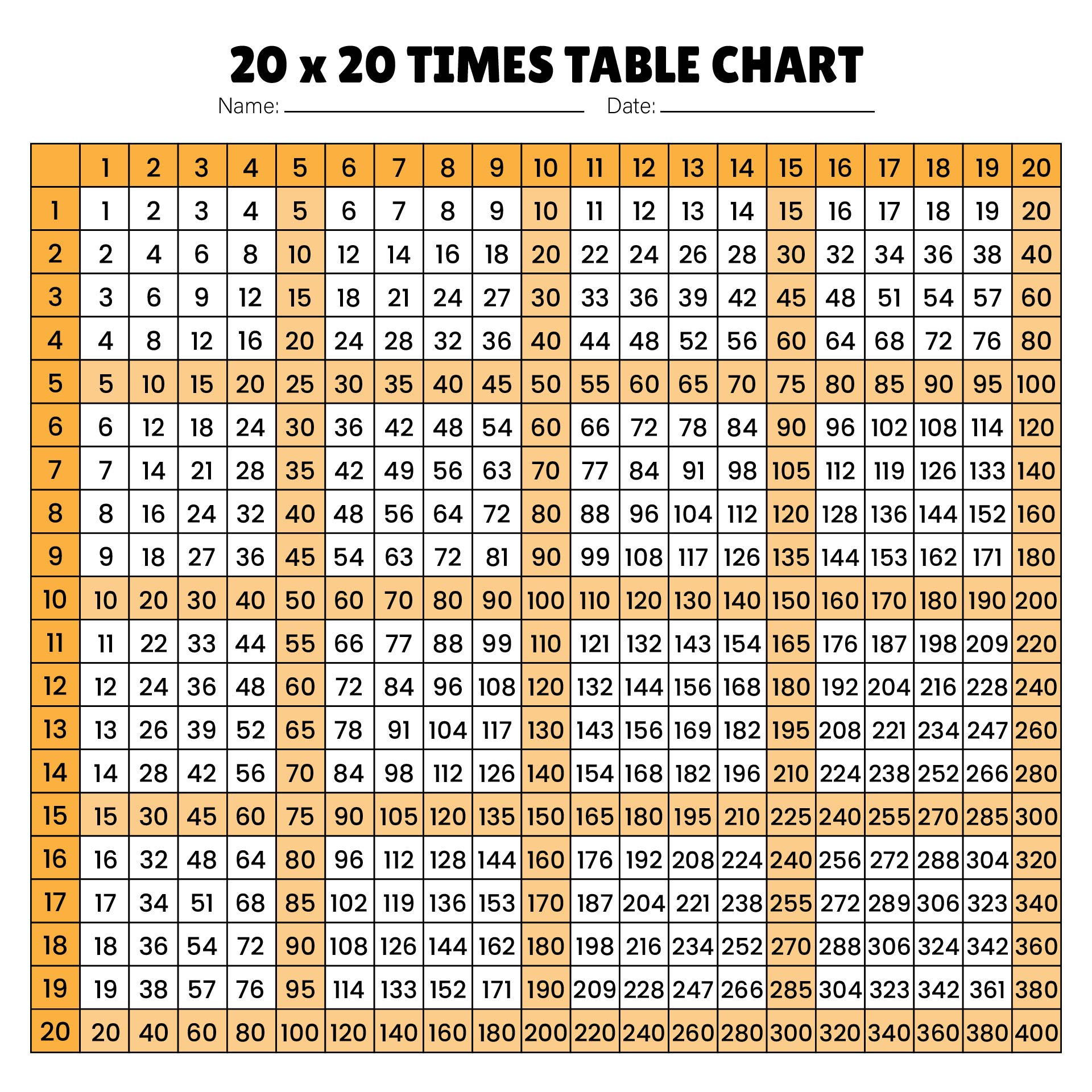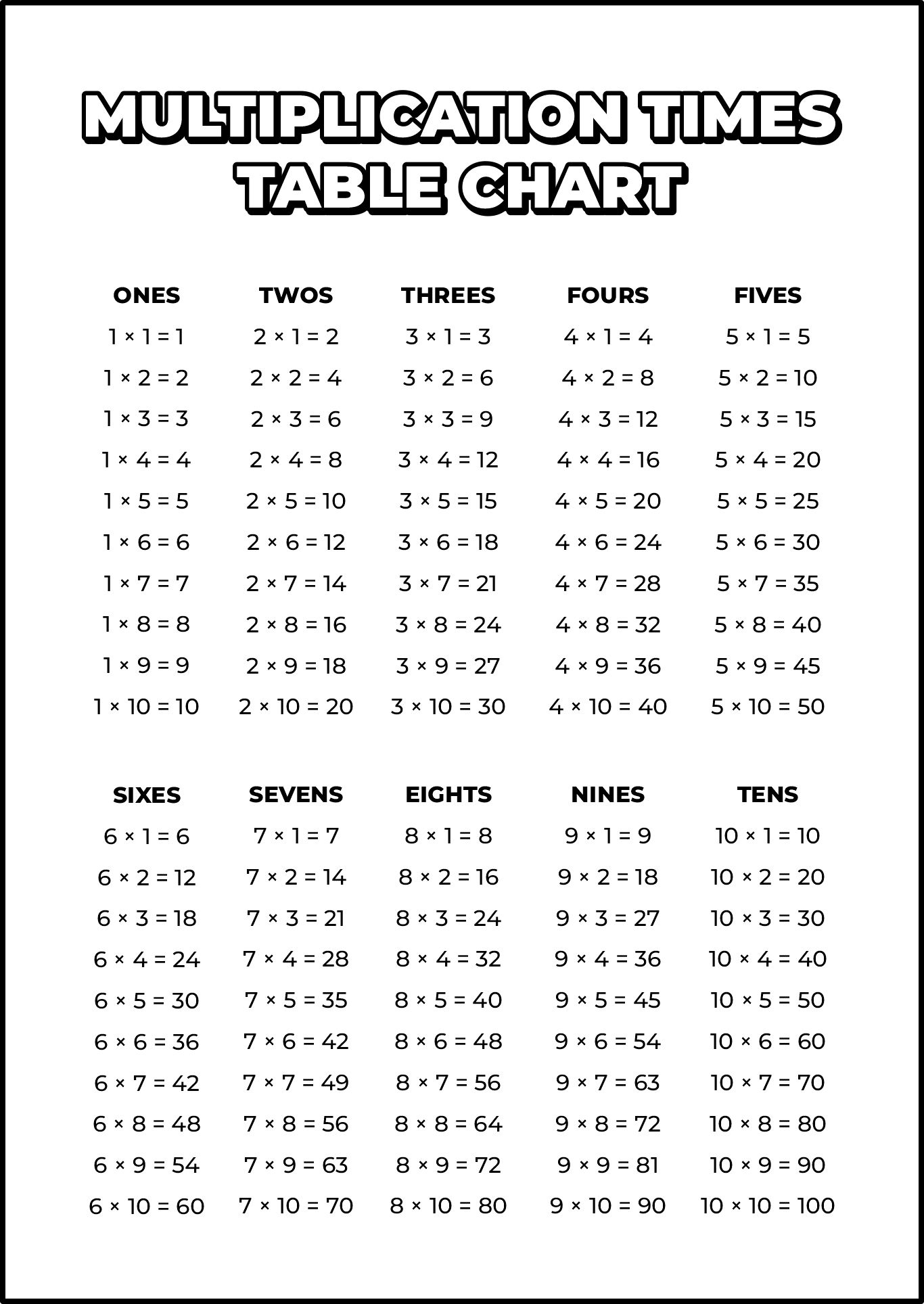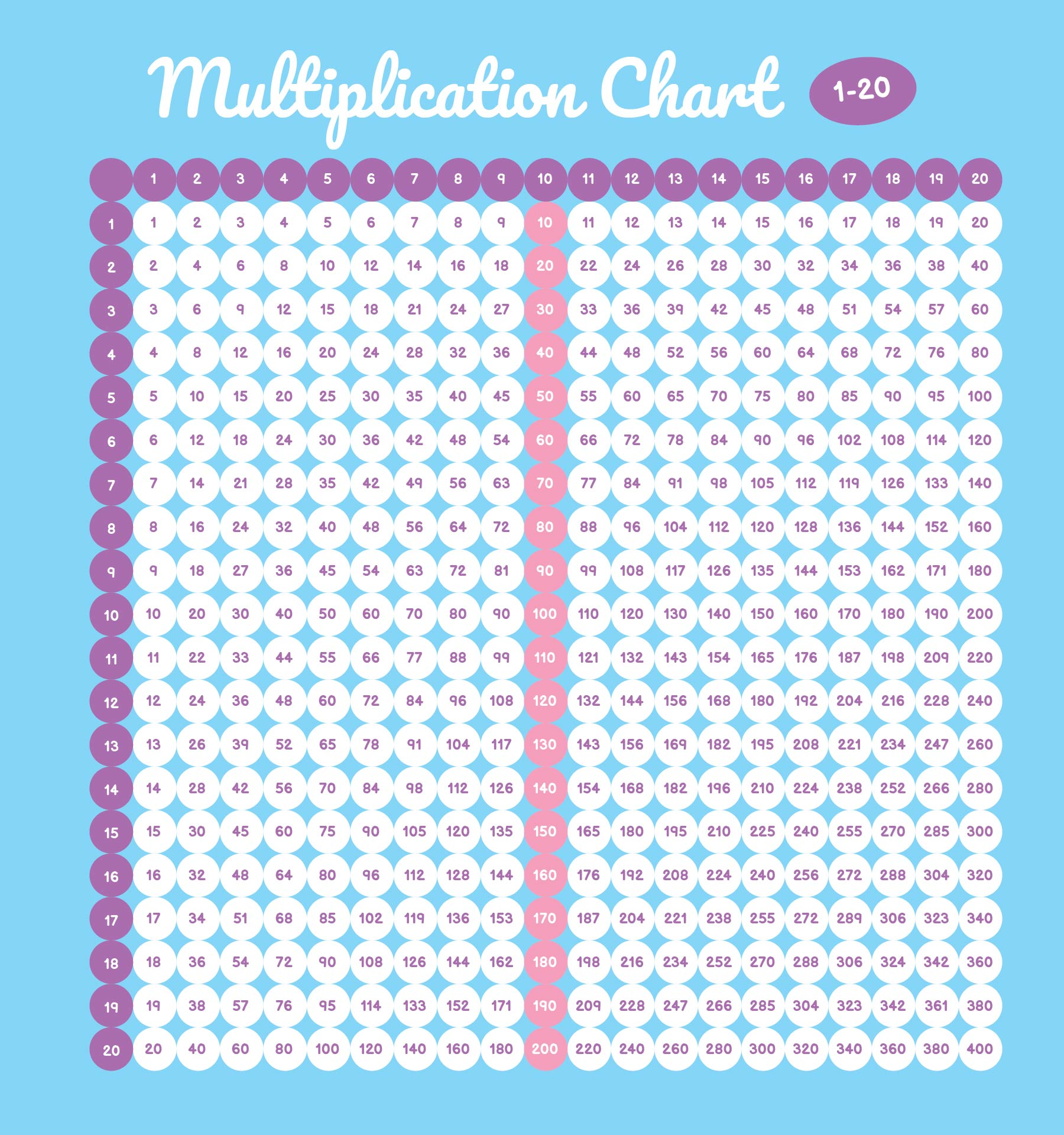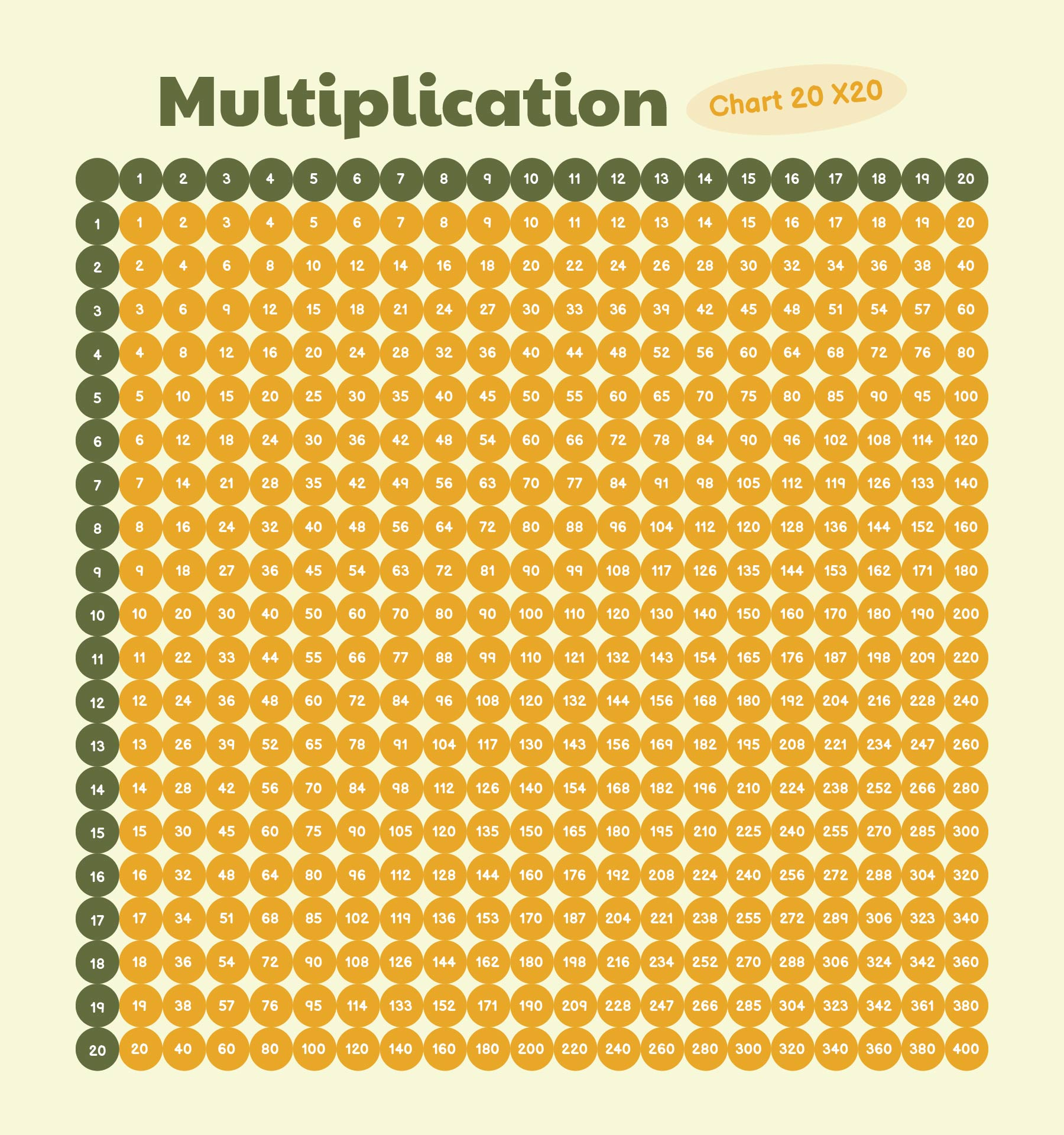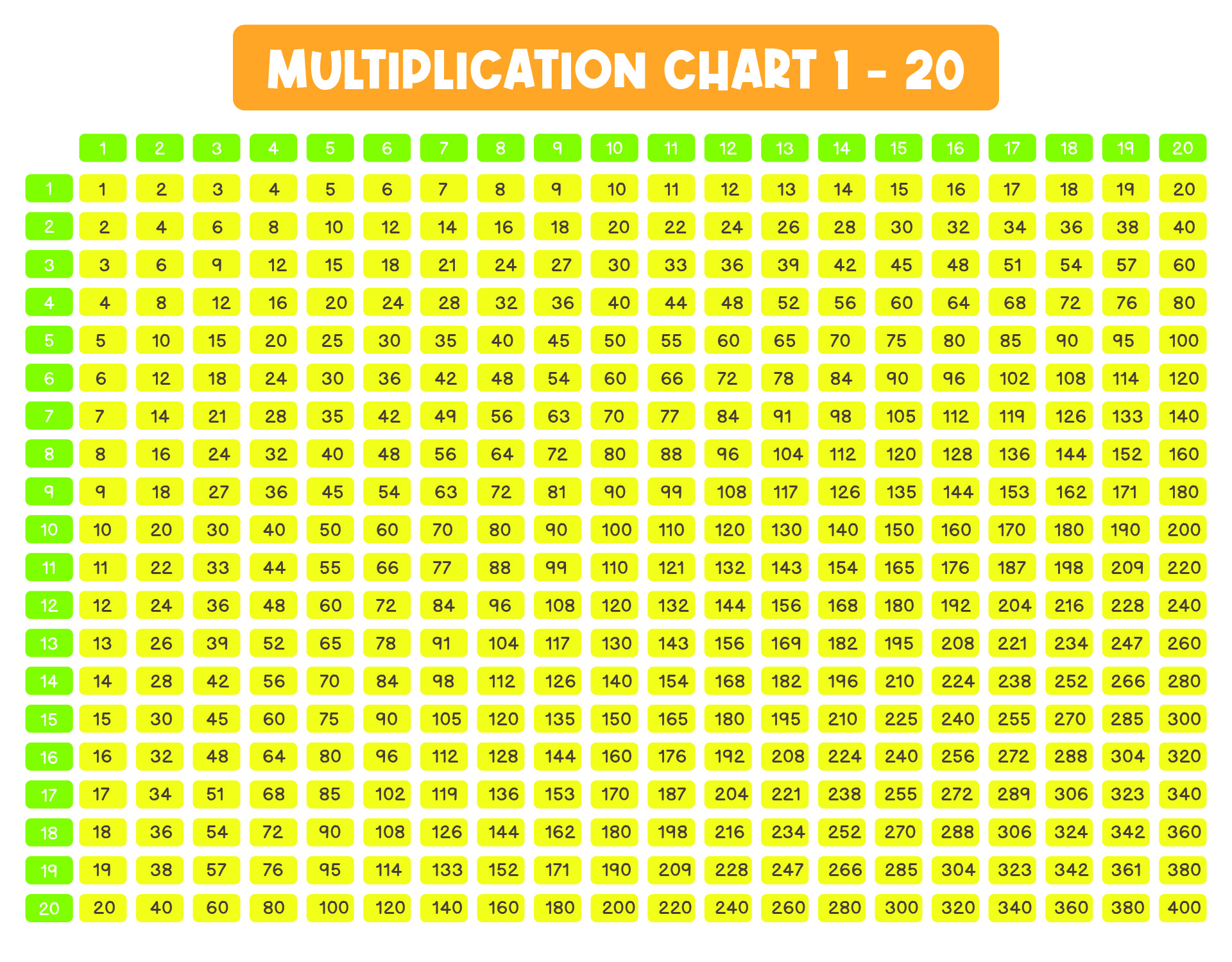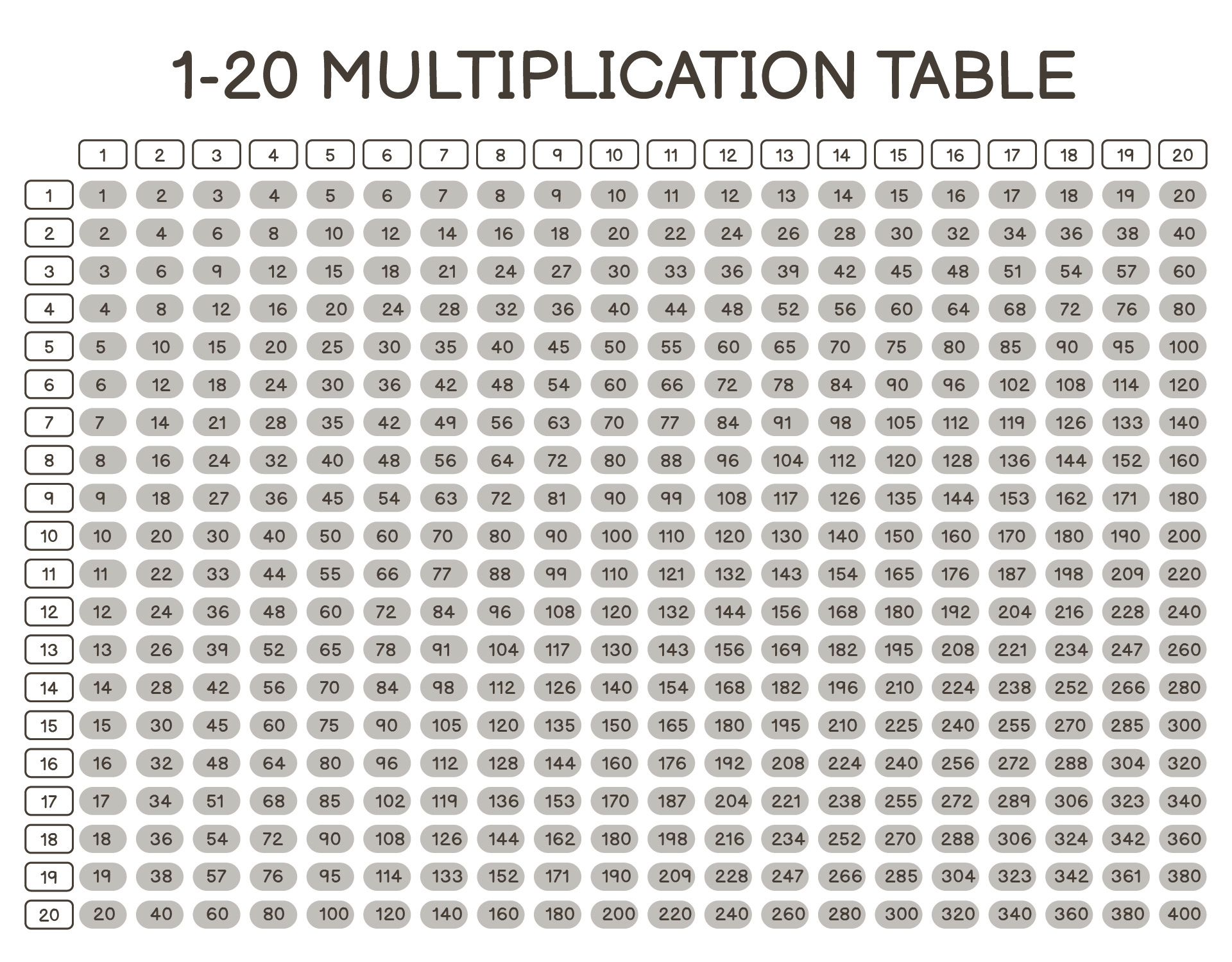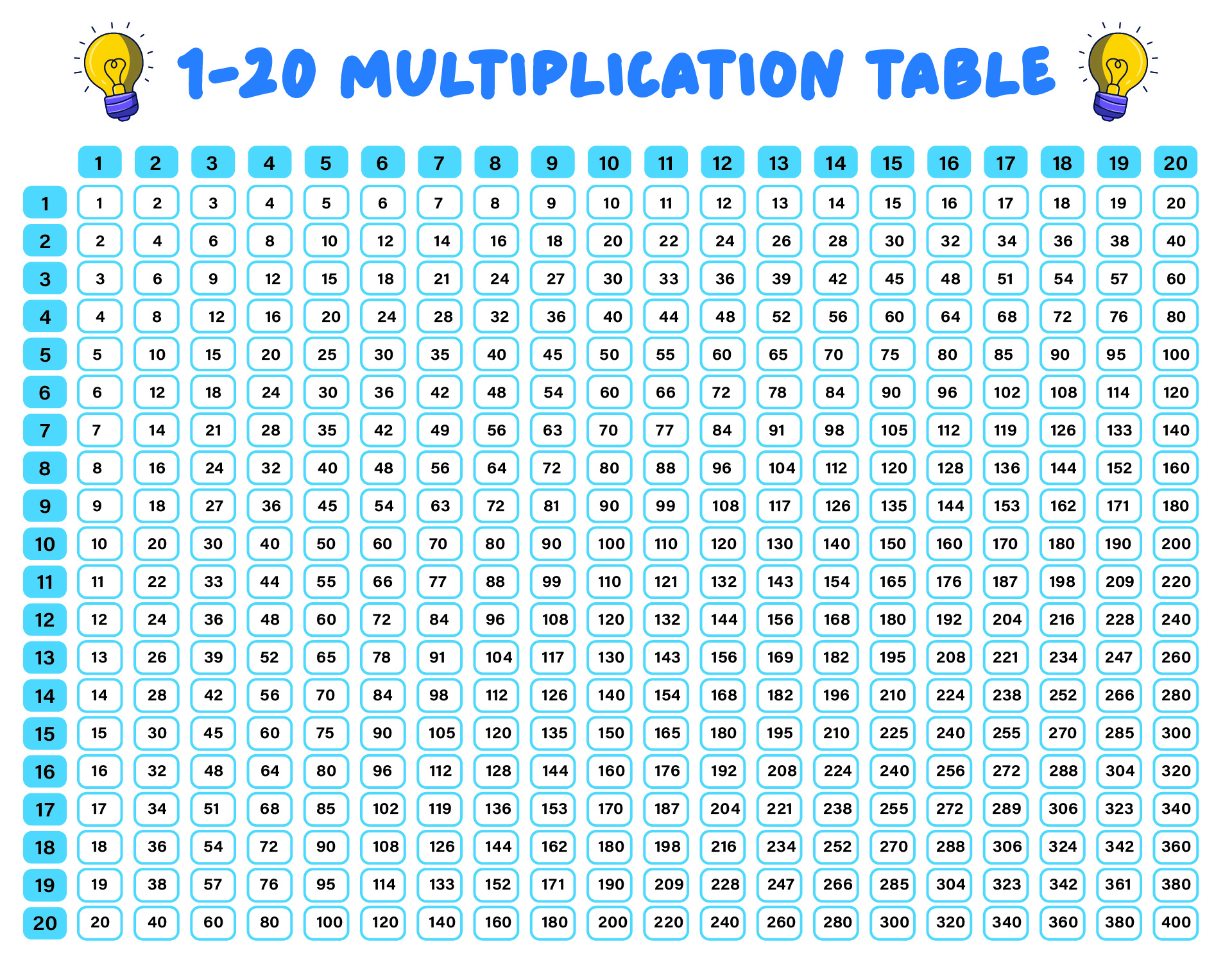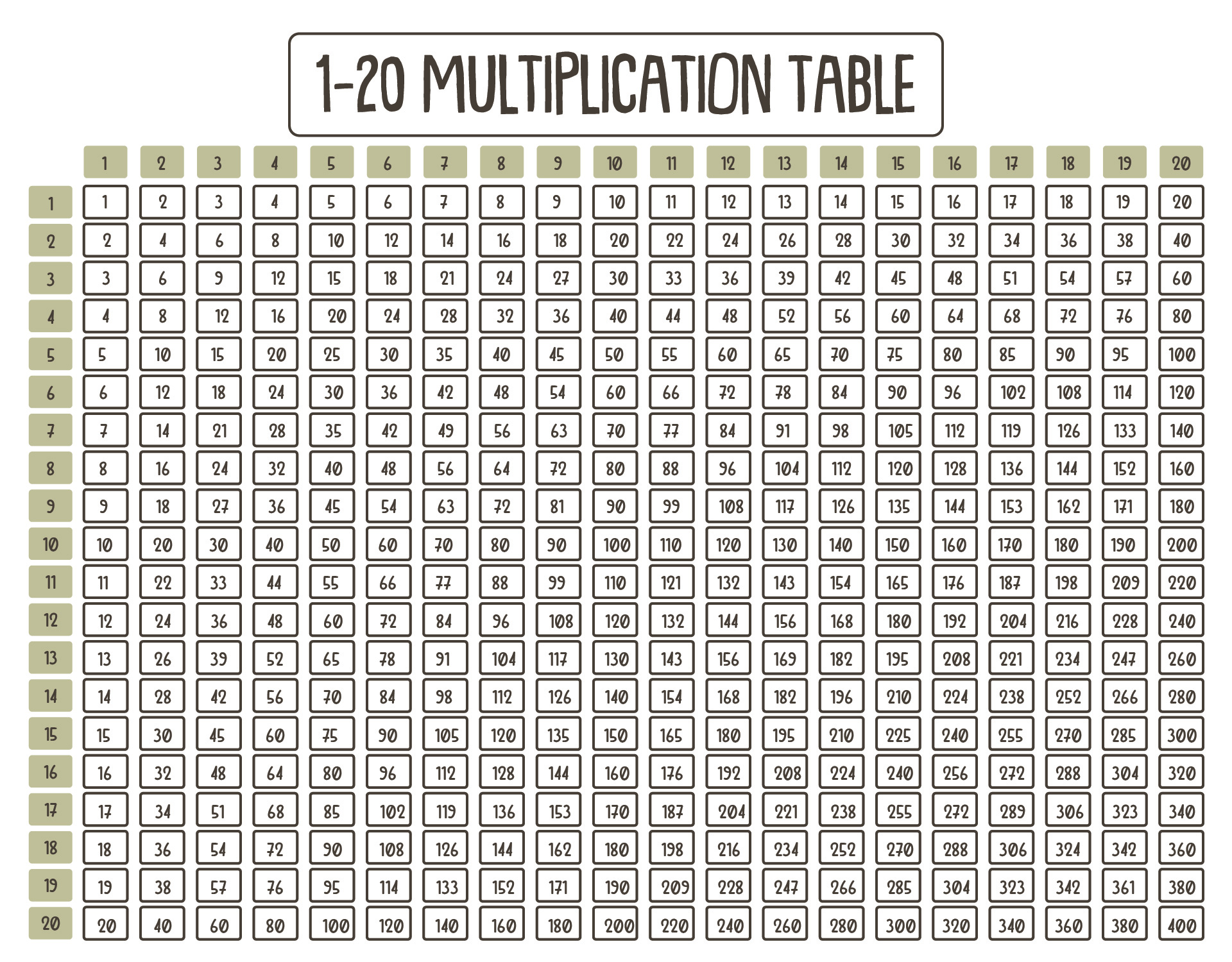### How do I teach my child the times tables?

It is a tricky thing when it comes to introducing and teaching multiplications, including using times tables multiplication chart 20 as a device. However, there are some effective ways to teach our child by using times tables.

Here are the effective ways:
1. Use a blank sheet of times table sheet
Time tables, including time tables multiplication chart 20 is considered as a good device to teach kids learning multiplication. Let them pick numbers to be multiplied. Then, let them fill inside the cell based on the position of the chosen number. Remember, a blank sheet of times table sheet contains so many cells. This is not designed to be completed in a single task. We can let them fill five cells first, then add more cells for the next day for a higher level of learning multiplication.
2. Let them understand the basic and easier multiplication first
It is believed that the easier multiplications are the multiplications of number 2, 5, and 10. It is considered so because the result of each multiplication has a similar pattern. Plus, when it comes to multiplication 2, they can simply count it with their fingers. If they are able to understand the concept of these kinds of multiplications and memorize the result, they can get advanced numbers, like the multiplications of 4 or 6.
3. Give tricks
Even though some people considered tricks as cheating, it is helpful for them to memorize the result accurately. For instance, when they want to understand the multiplication of 10, we can introduce and teach them that the result of this kind of multiplication is actually a multiplication of 1, but add the 0 after the multiplier. This helps them to follow the pattern so that they can recite the answers quickly.
4. Use a simple device
Besides the time tables multiplication chart 20, we can find other devices that can  be found inside our house. Yes, an analog clock and calculator. An analog clock is the best for skip counting and multiplication of number 5. It is because when a smaller and shorter needle points the certain numbers in the clock, they have a difference of five minutes. So, it is considered as a good device to learn the multiplication of 5. Then, we can also use a calculator. Indeed, this device is actually for counting, just like what the original function is. However, to utilize the function for multiplication usage, let them pick a certain number, then push + button, continue with pushing the same number, then let them push + again and again. The results are actually a multiplication and skip counting.